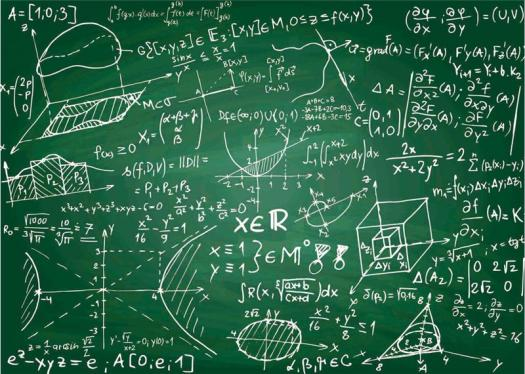# How Well Do You Know Polygonal Number?

Approved & Edited by ProProfs Editorial Team
At ProProfs Quizzes, our dedicated in-house team of experts takes pride in their work. With a sharp eye for detail, they meticulously review each quiz. This ensures that every quiz, taken by over 100 million users, meets our standards of accuracy, clarity, and engagement.
| Written by Hanero
H
Hanero
Community Contributor
Quizzes Created: 89 | Total Attempts: 39,536
Questions: 10 | Attempts: 139SettingsMathematics generally have polygonal numbers taking the form of a dot or if you close enough, the shape of a pebble.
Wherein the dots or pebbles are regarded as the alphas (units) and are one type of 2-dimensional figurate numbers.
​​​​Essentially, the total amount of dots/pebbles are numbers, and you arrange them into shapes.

• 1.

### What represents polygonal numbers often?

• A.

Fraction

• B.

Pebbles

• C.

Inequalities

• D.

Lines

B. Pebbles
Explanation
Polygonal numbers often represent pebbles.

Rate this question:

• 2.

### Which would you call the representation of polygonal numbers?

• A.

Units

• B.

Whole numbers

• C.

Fractions

• D.

Integers

A. Units
Explanation
The representation of polygonal numbers refers to numbers that can be arranged in the form of a polygon. Units, or numbers that represent a single entity or quantity, do not fit this description. Whole numbers, fractions, and integers can all be represented as polygonal numbers, but units cannot. Therefore, the correct answer is not units.

Rate this question:

• 3.

### How can you arrange a number of 10?

• A.

As A Trapezoid

• B.

As A Square

• C.

As A Rectangle

• D.

As A Triangle

D. As A Triangle
• 4.

### Into how many shapes can the number 36 be arranged?

• A.

Five shapes

• B.

Three shapes

• C.

Two shapes

• D.

A shape

C. Two shapes
Explanation
The number 36 can be arranged into two shapes.

Rate this question:

• 5.

### Which is the first polygonal number?

• A.

2

• B.

4

• C.

3

• D.

1

D. 1
Explanation
The first polygonal number refers to the first number in a sequence of numbers that can be arranged to form a regular polygon. In this case, the options given are 2, 4, 3, and 1. Among these options, 1 is the correct answer as it is the first polygonal number.

Rate this question:

• 6.

### Which of the following isn't a square number?

• A.

15

• B.

1

• C.

9

• D.

4

A. 15
Explanation
A square number is the result of multiplying an integer by itself. In this case, 15 is not a square number because there is no integer that can be multiplied by itself to equal 15. On the other hand, 1, 9, and 4 are all square numbers since they can be obtained by multiplying an integer by itself (1x1=1, 3x3=9, 2x2=4).

Rate this question:

• 7.

### Which of the following isn't a triangular number?

• A.

16

• B.

3

• C.

6

• D.

10

A. 16
Explanation
A triangular number is a number that can be represented as a triangle with dots. To determine if a number is triangular, we can use the formula n(n+1)/2, where n is a positive integer. Plugging in the given numbers, we find that 3, 6, and 10 can be represented as triangles with dots, but 16 cannot. Therefore, 16 is not a triangular number.

Rate this question:

• 8.

### Which of these is most important in knowing if a number is polygonal?

• A.

Size of circle in the polygon

• B.

Angles of the polygon

• C.

Size of the polygon

• D.

Sides of the polygon

D. Sides of the polygon
Explanation
The number of sides in a polygon is the most important factor in determining if a number is polygonal. A polygon is defined as a closed figure with straight sides, so the number of sides directly determines if a number can be represented as a polygon. The size of the circle in the polygon, the angles of the polygon, and the size of the polygon are all secondary factors that depend on the number of sides.

Rate this question:

• 9.

### What polygon is best used in determining polygonal numbers?

• A.

Octagon

• B.

Decagon

• C.

Heptagon

• D.

Hexagon

D. Hexagon
Explanation
Hexagon is the best polygon to determine polygonal numbers. A polygonal number is a number that can be represented by a regular polygon, where each side of the polygon represents a unit. Hexagon, with its six sides, provides a balanced and symmetrical shape that can easily represent polygonal numbers. Octagon, decagon, and heptagon have more or fewer sides, making them less suitable for this purpose.

Rate this question:

• 10.

### Which of these applies to polygonal numbers?

• A.

Henry's equation

• B.

Whole law

• C.

• D.

Pell's equationBack to top